Ex 14.2

Chapter 14 Class 10 Statistics
Serial order wise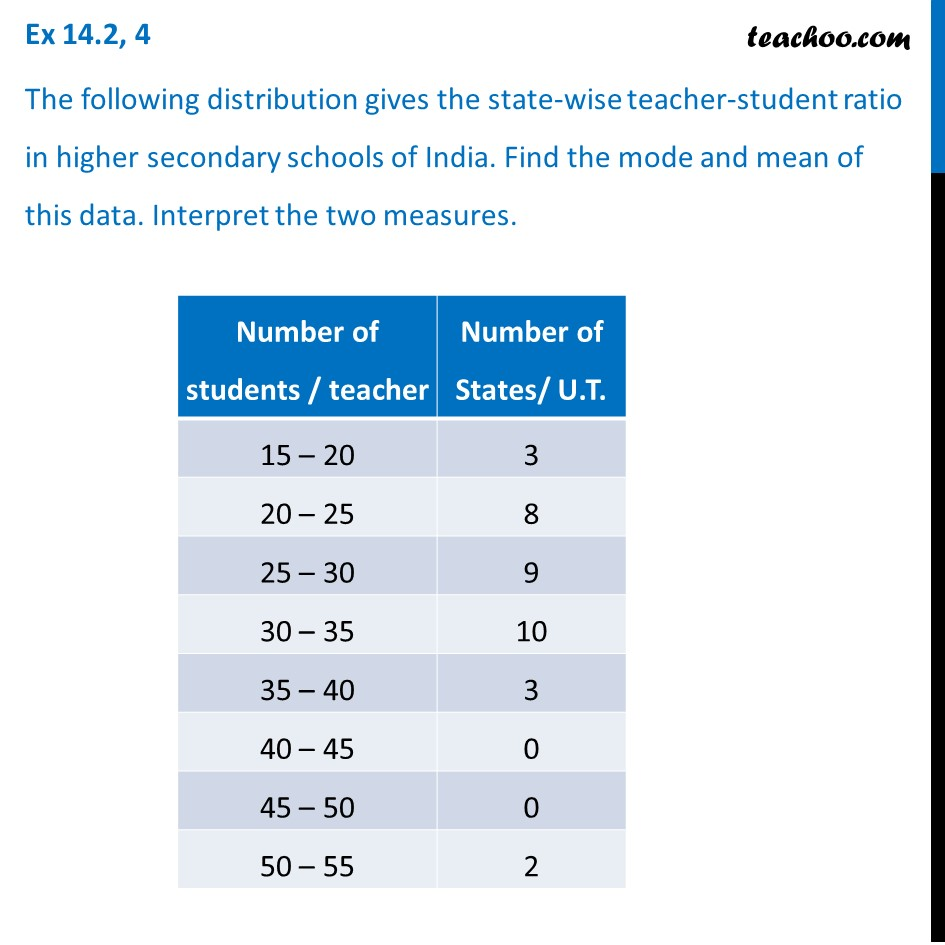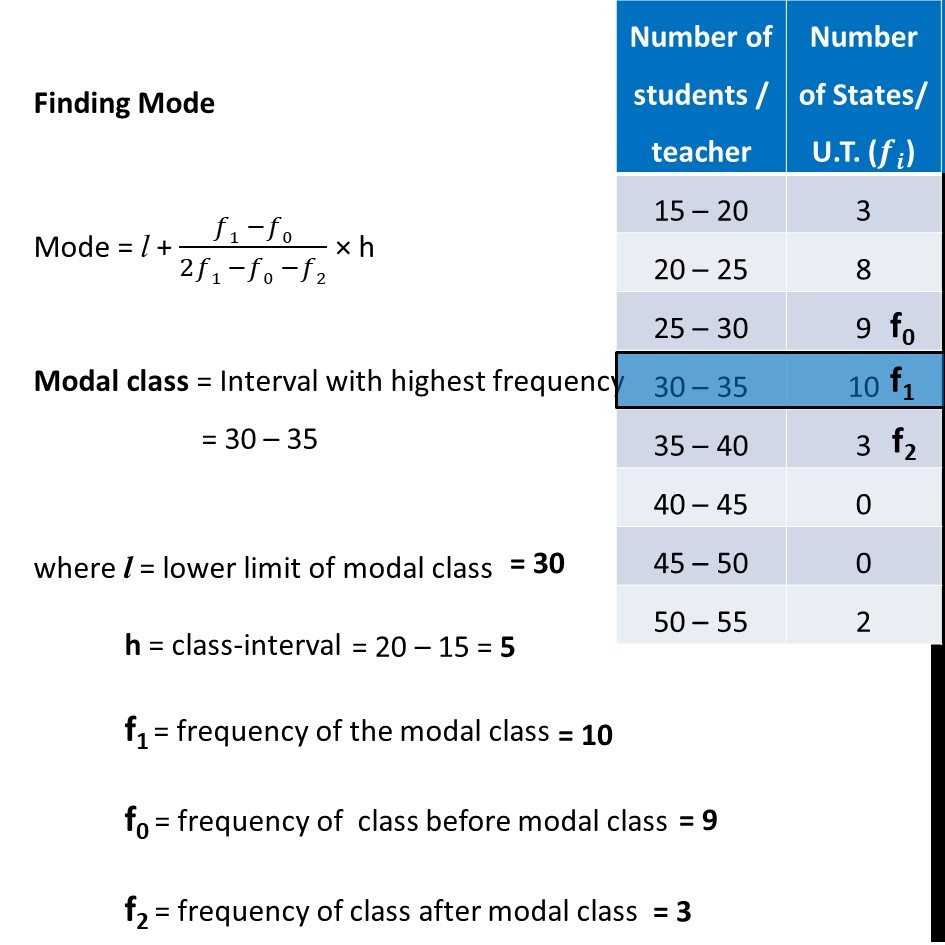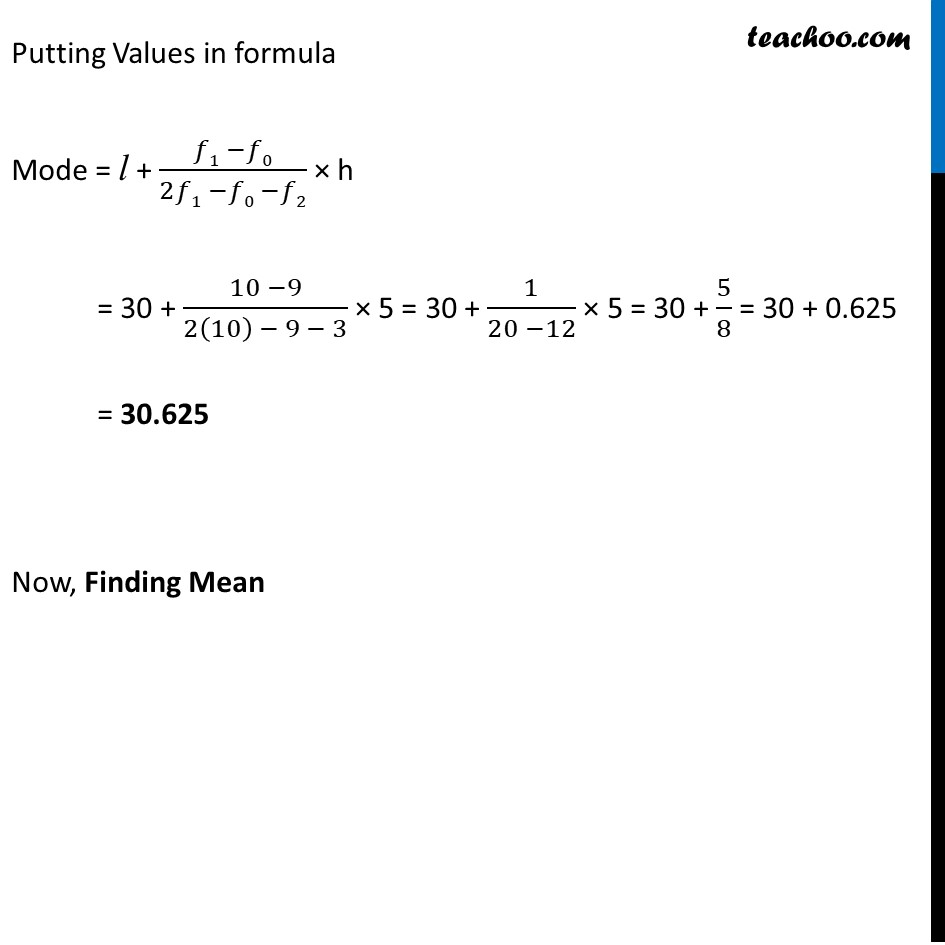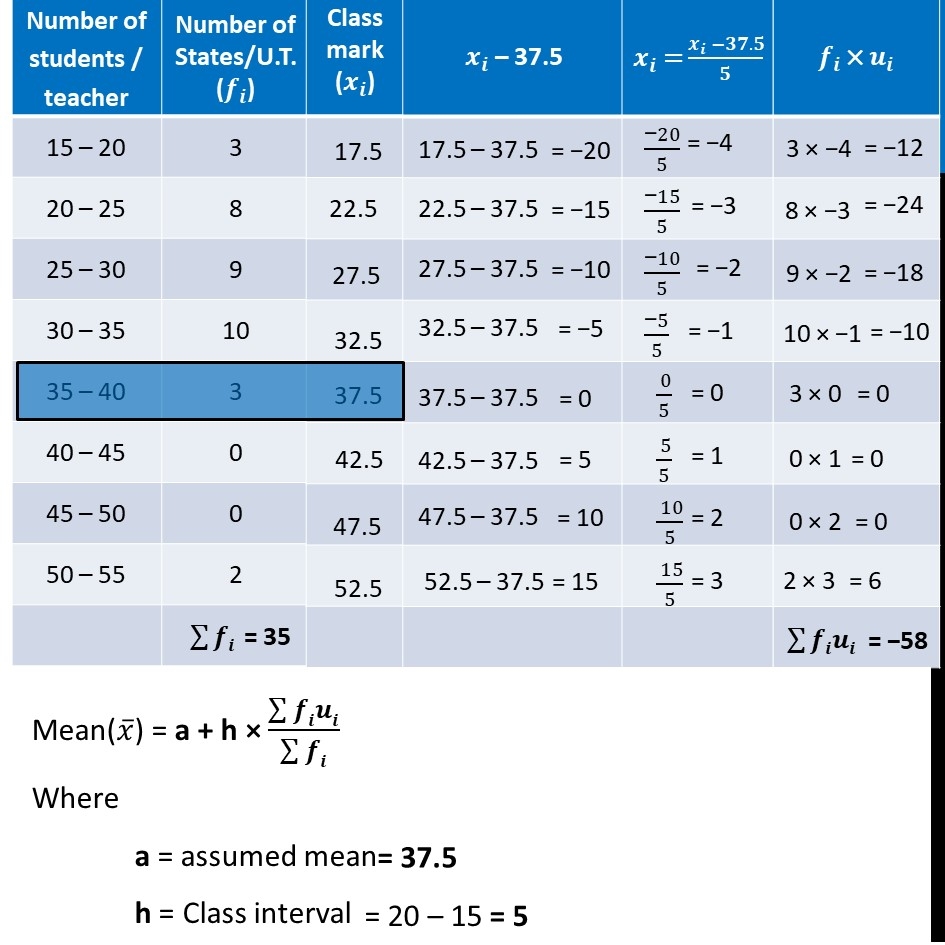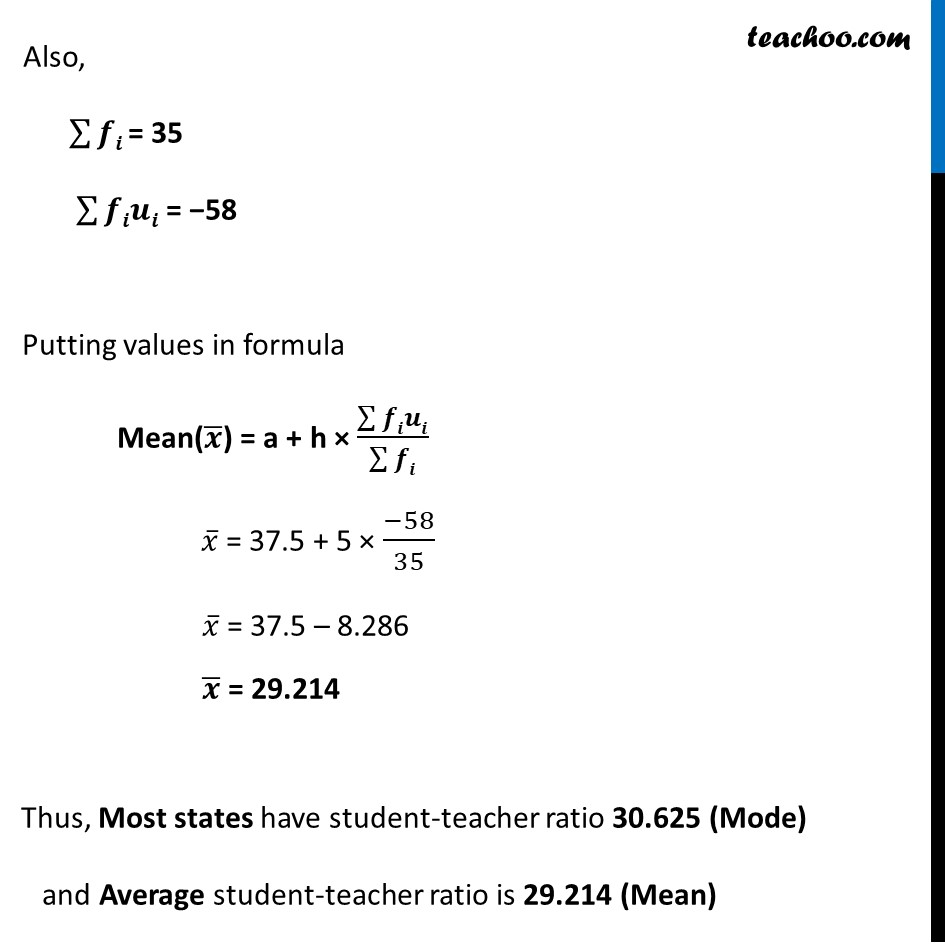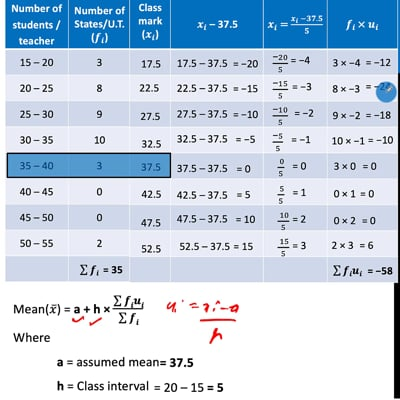This video is only available for Teachoo black users

Solve all your doubts with Teachoo Black (new monthly pack available now!)

### Transcript

Ex 14.2, 4 The following distribution gives the state-wise teacher-student ratio in higher secondary schools of India. Find the mode and mean of this data. Interpret the two measures. Finding Mode Mode = l + (𝑓1 −𝑓0)/(2𝑓1 −𝑓0 −𝑓2) × h Modal class = Interval with highest frequency = 30 – 35 where l = lower limit of modal class h = class-interval f1 = frequency of the modal class f0 = frequency of class before modal class f2 = frequency of class after modal class Putting Values in formula Mode = l + (𝑓1 −𝑓0)/(2𝑓1 −𝑓0 −𝑓2) × h = 30 + (10 −9)/(2(10) − 9 − 3) × 5 = 30 + 1/(20 −12) × 5 = 30 + 5/8 = 30 + 0.625 = 30.625 Now, Finding Mean Mean(𝑥 ̅) = a + h × (∑▒𝒇𝒊𝒖𝒊)/(∑▒𝒇𝒊) Where a = assumed mean h = Class interval = 20 – 15 = 5 Also, ∑▒𝒇𝒊 = 35 ∑▒𝒇𝒊𝒖𝒊 = −58 Putting values in formula Mean(𝒙 ̅) = a + h × (∑▒𝒇𝒊𝒖𝒊)/(∑▒𝒇𝒊) 𝑥 ̅ = 37.5 + 5 × (−58)/35 𝑥 ̅ = 37.5 – 8.286 𝒙 ̅ = 29.214 Thus, Most states have student-teacher ratio 30.625 (Mode) and Average student-teacher ratio is 29.214 (Mean)